Next: SLA_DBJIN - Decode String to B/J Epoch (DP)
Up: SUBPROGRAM SPECIFICATIONS
Previous: SLA_DAV2M - Rotation Matrix from Axial Vector

## SLA_DBEAR - Direction Between Points on a Sphere

ACTION:
Returns the bearing (position angle) of one point on a sphere relative to another (double precision).

CALL:
D = sla_DBEAR (A1, B1, A2, B2)

GIVEN:

 D A1,B1 spherical coordinates of one point A2,B2 D spherical coordinates of the other point

RETURNED:

 D sla_DBEAR bearing from first point to second

NOTES:
1.
The spherical coordinates are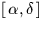,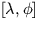etc., in radians.
2.
The result is the bearing (position angle), in radians, of point [A2,B2] as seen from point [A1,B1]. It is in the range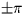. The sense is such that if [A2,B2] is a small distance due east of [A1,B1] the result is about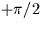. Zero is returned if the two points are coincident.
3.
If either B-coordinate is outside the range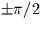, the result may correspond to the long way round''.
4.
The routine sla_DPAV performs an equivalent function except that the points are specified in the form of Cartesian unit vectors.Next: SLA_DBJIN - Decode String to B/J Epoch (DP)
Up: SUBPROGRAM SPECIFICATIONS
Previous: SLA_DAV2M - Rotation Matrix from Axial Vector

SLALIB --- Positional Astronomy Library